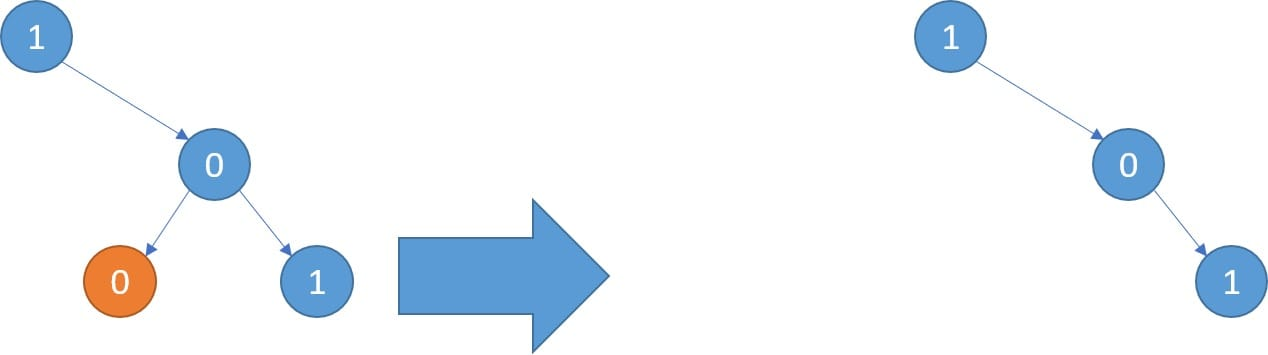# 814. Binary Tree Pruning

Reference: LeetCode
Difficulty: Medium

## Problem

We are given the head node root of a binary tree, where additionally every node’s value is either a 0 or a 1. Return the same tree where every subtree (of the given tree) not containing a 1 has been removed.

Recall that the subtree of a node X is X, plus every node that is a descendant of X.

Note:

• The binary tree will have at most 100 nodes.
• The value of each node will only be 0 or 1.

Example:## Analysis

Methods:

1. Recursion
• Prune children of the tree recursively. The only decision at each node is whether to prune the left child or the right child or not.
• containsOne(node): Whether the tree at this node contains 1, and it also prunes all subtrees not containing 1, e.g. node.left does not contain a 1, then we should prune it via node.left = null.
• Also, the current node’s value needs to be checked.
• Time: $O(N)$
• Space: $O(h)$

## Code

### Recursion

Note:

• This is the first version I wrote, but it turns out that containsOne(node) does a lot of repeated jobs.
• To improve, consider the traversal order. We can put the line 1 after visiting left subtree and right subtree. Therefore, traversal order sometimes is very important in performance or in reducing repeated calculations.

Change pruneTree to:

Change the line 1 & 2 to:

I try using a HashMap to cache those calculated nodes, but the runtime does not improve and even get worse.

#### Clean Code

Note:

• Can we do pruning in containsOne(node)?
• It is a postorder traversal. Can we do it in preorder way, which means checking the current node first? No! If root.val == 1, we cannot just return true, since we need to check the children.
• If root is null, we should return false. List all the base cases to see why it is the case.

CommentJunhao Wang
a software engineering cat